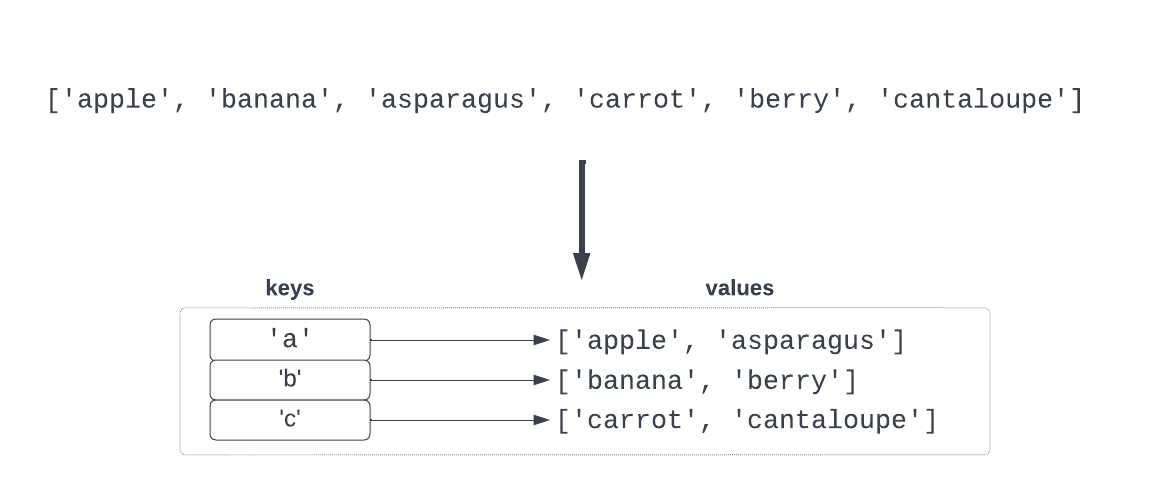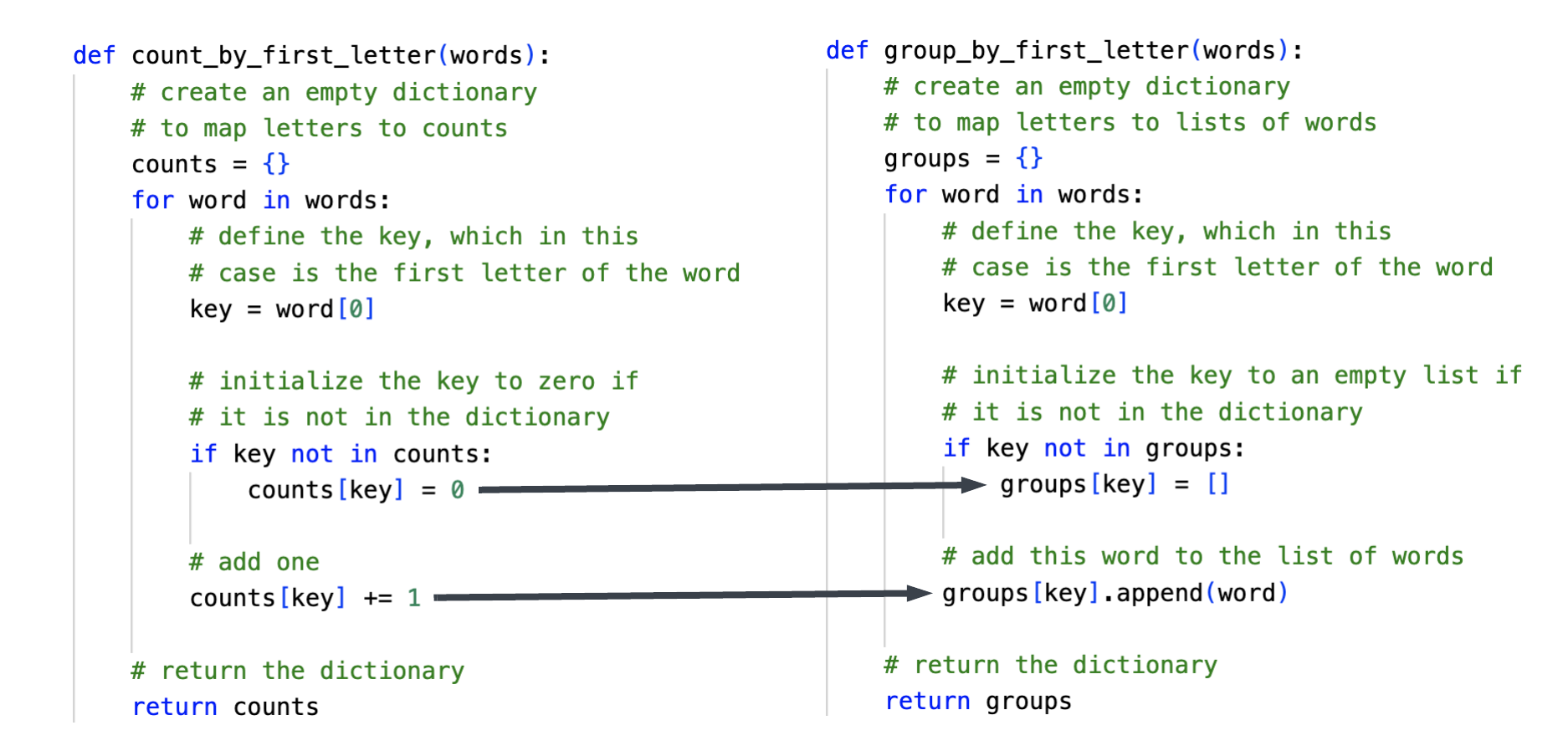Computer Science

# Grouping

One of the things you can do with a dictionary is group related items together. For example, you could take a list of words and group all of the words that start with the same first letter:We are going to show you a pattern for grouping lists of things into a dictionary.

## A dictionary that holds lists

If we wanted to manually put these words into a dictionary, we could do the following:

``````groups = {}

This maps the letter `a` to the list `['apple', 'avocado']`.

If we wanted to put words into the dictionary one at a time, we could do this:

``````groups['b'] = []
groups['b'].append('banana')``````

This maps the letter `b` to the list `[]` and then appends `banana` to this list. So in the end we have `b` mapped to `['banana']`.

## Grouping words one by one

You usually will not know in advance what words you want to group, so you will need to group them one by one. Here is a function that groups words based on their first letter:

``````def group_by_first_letter(words: list[str]) -> dict[str, list[str]]:
# create an empty dictionary to map letters to lists of words
groups = {}
for word in words:
# define the key, which in this case is the first letter of the word
key = word

# initialize the key to an empty list if it is not in the dictionary
if key not in groups:
groups[key] = []

# add this word to the list of words
groups[key].append(word)

# return the dictionary
return groups``````

Here is the pattern this follows:

• create an empty dictionary
• for each word
• define the key (e.g. the first letter in the word)
• initialize the dictionary for this key if needed, using an empty list
• add the word to the list of words for this key

You can see this in action in the file `group_by_first_letter.py`:

``````def group_by_first_letter(words: list[str]) -> dict[str, list[str]]:
"""
Group a list of words by their first letter.

words -> a list of strings

returns a dictionary that maps a letter to a list of words
"""
# create an empty dictionary to map letters to lists of words
groups = {}
for word in words:
# define the key, which in this case is the first letter of the word
key = word

# initialize the key to an empty list if it is not in the dictionary
if key not in groups:
groups[key] = []

# add this word to the list of words
groups[key].append(word)

# return the dictionary
return groups

def get_words() -> list[str]:
"""
Get a list of words from input
"""
words = []
while True:
word = input("Word: ")
if word == "":
break
words.append(word)

return words

def main():
words = get_words()
print(words)
groups = group_by_first_letter(words)
print(groups)

if __name__ == '__main__':
main()``````

This code gets a list of words from `input()`, prints the list of words, groups the words by first letter, then prints the groups. If you run it, you should see something like this:

``````Word: horse
Word: goat
Word: hamster
Word: guinea pig
Word: cow
Word:
['horse', 'goat', 'hamster', 'guinea pig', 'cow']
{'h': ['horse', 'hamster'], 'g': ['goat', 'guinea pig'], 'c': ['cow']}``````

## Grouping is a lot like counting

Grouping is really similar to counting! If we were counting words starting with the same letter, we would initialize the count to zero and then add one for each word. For grouping words starting with the same letter, we initialize the list to an empty list and then append each word to the list.You can see this in action in the file `count_by_first_letter.py`. It has nearly the same code, with small changes to make it count instead of group. If you run the code, you get something like this:

``````Word: horse
Word: goat
Word: hamster
Word: guinea pig
Word: cow
Word:
['horse', 'goat', 'hamster', 'guinea pig', 'cow']
{'h': 2, 'g': 2, 'c': 1}``````

## Grouping words by length

Can you modify `group_by_first_letter.py` to instead group by length? How would you do this?Here is a solution, which is in `group_by_length.py`:

``````def group_by_length(words: list[str]) -> dict[int, list[str]]:
"""
Group a list of words by their length.

words -> a list of strings

returns a dictionary that maps a letter to a list of words
"""
groups = {}
for word in words:
# key is the length of the word
key = len(word)

if key not in groups:
groups[key] = []

groups[key].append(word)

return groups

def get_words() -> list[str]:
"""
Get a list of words from input
"""
words = []
while True:
word = input("Word: ")
if word == "":
break
words.append(word)

return words

def main():
words = get_words()
print(words)
groups = group_by_length(words)
print(groups)

if __name__ == '__main__':
main()``````

Other than the name of the function and its documentation, the only thing that changes in `group_by_length()` is the key:

``key = len(word)``

The important part of grouping is picking the right key. Choose wisely.

If you run this program, you should see something like this:

``````Word: amazing
Word: totally
Word: fantastic
Word: just
Word: coolcool!
Word:
['amazing', 'totally', 'fantastic', 'just', 'coolcool!']
{7: ['amazing', 'totally'], 9: ['fantastic', 'coolcool!'], 4: ['just']}``````

## Using a tuple as a key

It is possible to use tuples as keys! For example, maybe you want to keep track of which classes are offered, based on combinations of day and time:

``````data = {
('Monday', '1pm'): 'CS 110',
('Tuesday', '2pm'): 'CS 235',
('Wednesday', '1pm'): 'CS 110',
('Thursday', '2pm'): 'CS 235',
('Friday', '1pm'): 'CS 110'
}``````

For example, the key `('Monday', '1pm')` maps to `'CS 110'`.

## First and last

This problem is a good example of using a tuple as as key. Can you modify `group_by_first_letter.py` so that it groups words by both their first and last letter?

Hint: To get the last letter of a word, you can use:

``last_letter = word[-1]``Here is a solution, which is in `group_by_first_and_last.py`:

``````def group_by_first_and_last_letter(words: list[str]) -> dict[tuple[str, str], list[str]]:
"""
Group a list of words by their first and last letters.

words -> a list of strings

returns a dictionary that maps a letter to a list of words
"""
# create an empty dictionary to map letters to lists of words
groups = {}
for word in words:
# the key is the first and last letter of the word
key = (word, word[-1])

# initialize the key to an empty list if it is not in the dictionary
if key not in groups:
groups[key] = []

# add this word to the list of words
groups[key].append(word)

# return the dictionary
return groups

def get_words() -> list[str]:
"""
Get a list of words from input
"""
words = []
while True:
word = input("Word: ")
if word == "":
break
words.append(word)

return words

def main():
words = get_words()
print(words)
groups = group_by_first_and_last_letter(words)
print(groups)

if __name__ == '__main__':
main()``````

Again, the only line that has changed here is the key, which this time is a tuple:

``key = (word, word[-1])``

If you run this program, you should see something like:

``````Word: awesome
Word: great
Word: apple
Word: goat
Word: wow
Word: willow
Word:
['awesome', 'great', 'apple', 'goat', 'wow', 'willow']
{('a', 'e'): ['awesome', 'apple'], ('g', 't'): ['great', 'goat'], ('w', 'w'): ['wow', 'willow']}``````

## Group by number of vowels

Here is one last example. Can you change this same code so that it groups by the number of vowels in the word?Here is a solution, which is in `group_by_number_of_vowels.py`:

``````def count_vowels(word: str) -> int:
total = 0
for c in word.lower():
if c in 'aeiou':
total += 1

def group_by_number_of_vowels(words: list[str]) -> dict[int, list[str]]:
"""
Group a list of words by the number of vowels they contain.

words -> a list of strings

returns a dictionary that maps a letter to a list of words
"""
# create an empty dictionary to map letters to lists of words
groups = {}
for word in words:
# the key is the first and last letter of the word
key = count_vowels(word)

# initialize the key to an empty list if it is not in the dictionary
if key not in groups:
groups[key] = []

# add this word to the list of words
groups[key].append(word)

# return the dictionary
return groups

def get_words() -> list[str]:
"""
Get a list of words from input
"""
words = []
while True:
word = input("Word: ")
if word == "":
break
words.append(word)

return words

def main():
words = get_words()
print(words)
groups = group_by_number_of_vowels(words)
print(groups)

if __name__ == '__main__':
main()``````

Notice that this time we need a function to compute the key. We wrote a function called `count_vowels()` that takes a word and returns the number of vowels in the word. We can use the return value of this function for the key.

You should see something like this if you run the program:

``````Word: just
Word: really
Word: smashing
Word: great
Word: job
Word:
['just', 'really', 'smashing', 'great', 'job']
{1: ['just', 'job'], 2: ['really', 'smashing', 'great']}``````

## Closing words

In all of these examples, we got the words to group from `input()`. You might in some cases get the words from a regular file, or you might get rows of data from a CSV file. If you decompose the problem so that you first get the data and then group it, then you should be able to follow the pattern we have shown here.